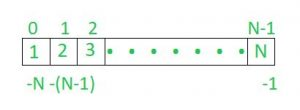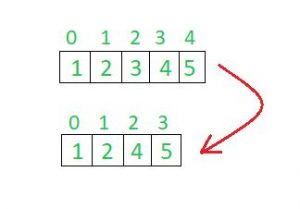# Internal working of list in Python

Introduction to Python lists :
Python lists are internally represented as arrays. The idea used is similar to implementation of vectors in C++ or ArrayList in Java. The costly operations are inserting and deleting items near the beginning (as everything has to be moved). Insert at the end also becomes costly if preallocated space becomes full.

We can create a list in python as shown below.

Example:

 `list1 ``=` `[``1``, ``2``, ``3``, ``4``] `

We can access each element of a list in python by their assigned index. In python starting index of list sequence is 0 and ending index is (if N elements are there) N-1.Also as shown in above array lists also have negative index starting from -N (if N elements in the list) till -1.

Viewing the elements of List in Python :
Individual items of a list can be accessed through their indexes as done in below code segment.

 `list1 ``=` `[``1``, ``2``, ``3``, ``4``] ` ` `  `# for printing only one item from a list ` `print``(list1[``1``]) ` ` `  `# to print a sequence of item in a list ` `# we use ':' value before this is starting  ` `# and value after that tells ending of sequence ` `print``(list1[``1``:``4``]) ` ` `  `# accessing through negative index ` `print``(list1[``-``1``]) `

Assigning and Accessing data:
For creating a list we need to specify the elements inside square brackets ‘[]’ and then give it a name. Whenever you want to access the list elements then use this list name and index of element you want to show.
Each element in list is assigned an index in positive indexing we have index from 0 to end of the list and in negative indexing we have index from -N(if elemts are N) till -1.
As shown in above examples the work of accessing elements is manual. We can also access or assign elements through loops.

 `# assigning elements to list ` `list1 ``=``[] ` `for` `i ``in` `range``(``0``, ``11``): ` `     ``list1.append(i) ` ` `  `# accessing elements from a list ` `for` `i ``in` `range``(``0``, ``11``): ` `     ``print``(list1[i]) `

Updating list:
We can update already assigned elements to the list and also can append one element at a time to your list.Even you can extend your list by adding another list to current list.
The above task can be performed as follows.

 `list1 ``=``[``1``, ``2``, ``3``, ``4``] ` ` `  `# updating ` `list1[``2``]``=` `5` `print``(list1) ` ` `  `# appending ` `list1.append(``6``) ` `print``(list1) ` ` `  `# extending ` `list1.extend([``1``, ``2``, ``3``]) ` `print``(list1) `

Note: append() and extend() are built in methods in python for lists.

Deleting elements of list :
We can delete elements in lists by making use of del function. In this you need to specify the position of element that is the index of the element and that element will be deleted from the list and index will be updated.In above shown image the element 3 in index 2 has been deleted and after that index has been updated.

 `list1 ``=` `[``1``, ``2``, ``3``, ``4``, ``5``] ` `print``(list1) ` ` `  `# deleting element ` `del` `list1[``2``] ` `print``(list1) `

Time Complexities of Operations

 Operation Average Case Amortized Worst Case Copy O(n) O(n) Append O(1) O(1) Pop last O(1) O(1) Pop intermediate O(k) O(k) Insert O(n) O(n) Get Item O(1) O(1) Set Item O(1) O(1) Delete Item O(n) O(n) Iteration O(n) O(n) Get Slice O(k) O(k) Del Slice O(n) O(n) Set Slice O(k+n) O(k+n) Extend O(k) O(k) Sort O(n log n) O(n log n) Multiply O(nk) O(nk) x in s O(n) O(n) min(s), max(s) O(n) O(n) Get Length O(1) O(1)

Source : Python Wiki

Python list and its operations.

My Personal Notes arrow_drop_upCheck out this Author's contributed articles.

If you like GeeksforGeeks and would like to contribute, you can also write an article using contribute.geeksforgeeks.org or mail your article to contribute@geeksforgeeks.org. See your article appearing on the GeeksforGeeks main page and help other Geeks.

Please Improve this article if you find anything incorrect by clicking on the "Improve Article" button below.

Article Tags :
Practice Tags :

Be the First to upvote.

Please write to us at contribute@geeksforgeeks.org to report any issue with the above content.